# KVPY-SX 2018 Math Paper with Solutions

KVPY-SX 2018 paper with solutions Maths is a perfect resource for those who are preparing for KVPY examination. The first step towards the KVPY Exam Preparation is to solve the Previous Year Question Papers. Along with the question paper, the answer key has also been provided here. Solving the previous years’ papers will give you an idea about the nature and pattern of questions asked in the exam. It will help the students to get a clear idea about the amount of preparation required to face the exam.

### KVPY-SX 2018 - Maths

Question 1: Suppose A = $\begin{bmatrix} a & b\\ c & d \end{bmatrix}$ is a real matrix with nonzero entries, ad – bc = 0, and A2 = A. Then a + d equals

1. a. 1
2. b. 2
3. c. 3
4. d. 4

Solution:

A2 = A (given)

$\begin{bmatrix} a & b\\ c & d \end{bmatrix}\begin{bmatrix} a & b\\ c & d \end{bmatrix} = \begin{bmatrix} a & b\\ c & d \end{bmatrix}$

Using matrix multiplication (row x column method)

$\begin{bmatrix} a^2+bc & ab+bd\\ ca+dc & cb+d^2 \end{bmatrix}$

On comparing both sides.

ab + bd = b (since b ≠ 0)

a + d = 1

Question 2: On any given arc of positive length on the unit circle |z| = 1 in the complex plane.

1. a. there need not be any root of unity
2. b. there lies exactly one root of unity
3. c. there are more than one but finitely many roots of unity
4. d. there are infinitely many roots of unity

Solution:

|z| = 1 (given)

zn = 1

Using concept of nth root of unity,

z = e[2ik𝛑/n], where k ∈ {0, 1, 2, …., n – 1}

put k = 0 ⇒ z = e0 ⇒ z = 1

put k = 1 ⇒ z = e[2i𝛑/n]

put k = 2 ⇒ z = e[4i𝛑/n]

All these roots of unity will lie on circle |z| = 1.

So, we can say, there are infinitely many roots of unity.

Question 3: For 0 < θ < 𝛑 / 2 , four tangents are drawn at the four points (± 3 cos θ, ± 2 sin θ) to the ellipse (x2 / 9) + (y2 / 4) = 1. If A(θ) denotes the area of the quadrilateral formed by these four tangents, the minimum value of A(θ) is

1. a. 21
2. b. 24
3. c. 27
4. d. 30

Solution:

(x2 / 9) + (y2 / 4) = 1

Using parametric coordinate of the ellipse (a cos θ, b sin θ) = (3 cos θ, 2 sin θ)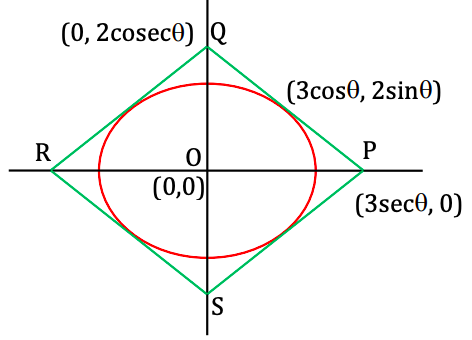Equation of tangent at point (3 cos θ, 2 sin θ) is T = 0 {T = xx1 / a2 + yy1 / b2 = 1}

PQ: [3x cos θ / 9] + [2y sin θ / 4] = 1

PQ: [x cos θ / 3] + [y sin θ / 2] = 1

At x = 0 ⇒ y = 2 cosec θ

At y = 0 ⇒ x = 3 sec θ

Area of quadrilateral A (θ) = 4 × Area of △OPQ

= 4 × (1 / 2) × Base (OP) × Height (OQ)

= 4 × (1 / 2) × 3 sec θ . 2 cosec θ

= 12 / [sin θ cos θ]

= 24 / sin 2θ

Minimum A(θ) = 24 / (sin 2θ)max = 24 / 1 = 24

Question 4: Let S = {x ∈ R: cos(x) + cos (√2x) < 2}. Then

1. a. S = ɸ
2. b. S is a non-empty finite set
3. c. S is an infinite proper subset of R\{0}
4. d. S = R -{0}

Solution:

We know cosx + cos √2x will always be less than 2 except

cos x = 1 & cos √2 x = 1

x = 2n𝛑 & √2 x = 2n𝛑

x = √2n𝛑

both are simultaneously possible at x = 0

So, S = R – {0}

Question 5: On a rectangular hyperbola x2 – y2 = a2, a > 0, three points A, B, C are taken as follows: A = (–a, 0); B and C are placed symmetrically with respect to the x-axis on the branch of the hyperbola not containing A. Suppose that the triangle ABC is equilateral. If the side-length of the triangle ABC is ka, then k lies in the interval

1. a. (0, 2]
2. b. (2, 4]
3. c. (4, 6]
4. d. (6, 8]

Solution:

Let points B and C on hyperbola x2 – y2 = a2

B ≡ (a sec θ, a tan θ) & C ≡ (a sec θ, –a tan θ)

{Using parameter coordinates of hyperbola}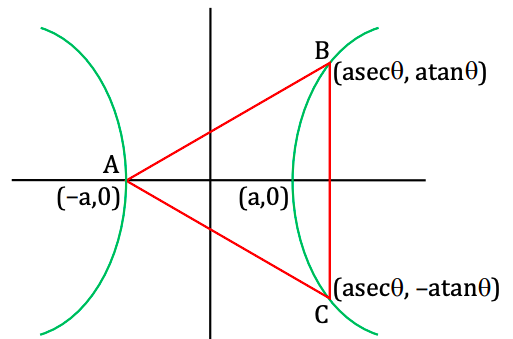Since △ABC is equilateral, AB = BC

On squaring both sides

(AB)2 = (BC)2

(a sec θ + a)2 + (a tan θ)2 = (2a tan θ)2

sec2 θ + 1 + 2 sec θ + tan2 θ = 4 tan2 θ

1 + tan2 θ + 1 + 2 sec θ + tan2 θ = 4 tan2 θ

2 + 2 sec θ = 4 tan2 θ

sec2 θ – sec θ – 2 = 0 {tan2 θ = sec2 θ – 1}

(sec θ – 2) (sec θ + 1) = 0

sec θ = 2 or sec θ = –1 (not possible, because for sec θ = –1 B and C will co-inside with A)

(1 / cos θ) = 2

cos θ = 1 / 2

θ = 𝛑 / 3 (put in parametric coordinates of B & C)

So, points B and C are B ≡ (2a, √3a) & C ≡ (2a, – √3 a)

BC = 2 √3a = side length

On comparing with question ka = 2√3a

k = 2√3

Question 6: The number of real solutions x of the equation cos2 (x sin (2x)) + (1 / [1 + x2] = cos2x + sec2x is:

1. a. 0
2. b. 1
3. c. 2
4. d. infinite

Solution:

cos2 (x sin (2x)) + (1 / [1 + x2]) = cos2x + sec2x

LHS: cos2 (x sin(2x)) + (1 / [1 + x2])

cos2 (x sin2x) ∈ [0, 1] .....(i) cos2 θ ∈ [0, 1]

0 ≤ x2 < ∞

1 ≤ x2 + 1 < ∞

1 ≥ (1 / [1 + x2]) > 1 / ∞ = 0

(1 / [1 + x2]) ∈ (0, 1] .....(ii)

0 < cos2 (x sin (2x)) + (1 / [1 + x2]) ≤ 2

RHS: cos2x + sec2x

cos2x + (1 / cos2x)

using AM ≥ GM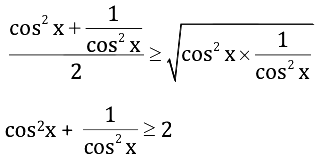LHS ≤ 2

RHS ≥ 2

LHS & RHS both will be equal only when x = 0.

Number of solutions = 1.

Question 7: Let x2 / a2 + y2 / b2 = 1, a > b, be an ellipse with foci F1 and F2. Let AO be its semi-minor axis, where O is the centre of the ellipse. The lines AF1 and AF2, when extended, cut the ellipse again at points B and C respectively. Suppose that the triangle ABC is equilateral. Then the eccentricity of the ellipse is

1. a. 1 / √2
2. b. 1 / √3
3. c. 1 / 3
4. d. 1 / 2

Solution: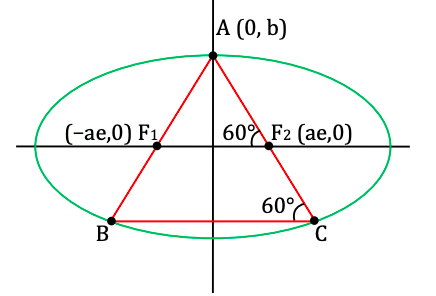mAF2 = tan 120°

[b - 0] / [0 - ae] = tan 120o = -√3 [As m = [y2 - y1] / [x2 - x1]]

b / ae = √3

On squaring both sides

b2 / a2e2 = 3 {using e = √1 - [b2 / a2]}

a2 [1 - e2] / a2e2 = 3

1 - e2 = 3e2

e2 = 1 / 4

taking square root both sides

e = 1 / 2

Question 8: Let a = cos 1° and b = sin 1°. We say that a real number is algebraic if it is a root of a polynomial with integer coefficients. Then

1. a. a is algebraic but b is not algebraic
2. b. b is algebraic but a is not algebraic
3. c. both a and b are algebraic
4. d. neither a nor b is algebraic

Solution:

If cos 1° = a + √b (a, b ∈ Q)

Then it will be root of a quadratic equation whose other root is a – √b

x2 – (sum of roots)x + Product of roots = 0

x2 – (a + √b + a – √b)x + (a – √b) (a +√ b) = 0

x2 – 2ax + (a2 – b) = 0 (real integers) {Using (a – b)(a + b) = a2 – b2}

cos 1° is algebraic

Similarly

sin 1° is also algebraic.

Question 9: A rectangle with its sides parallel to the x-axis and y-axis is inscribed in the region bounded by the curves y = x2 – 4 and 2y = 4 – x2. The maximum possible area of such a rectangle is closest to the integer

1. a. 10
2. b. 9
3. c. 8
4. d. 7

Solution: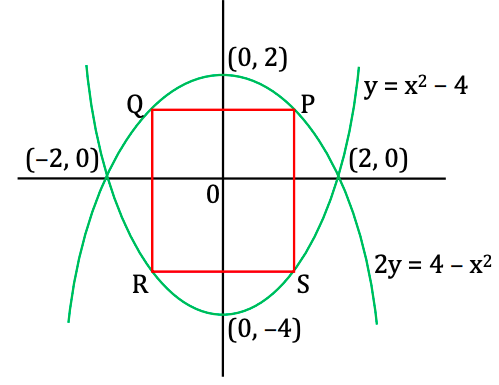Let points on curve 2y = 4 – x2 is

P [x1, 2 - (x12 / 2)]; Q [-x1, 2 - (x12 / 2)]

Points on curve y = x2 – 4 is

S (x1, x12 – 4); R (–x1, x12 – 4)
Area PQRS is A ≡ (PQ × PS) {Area of rectangle = length × width}

A = 2x1 * [2 - (x12 / 2) - (x12 - 4)]

A = 2x1 * [6 - (3 / 2) x12]

A = 12x1 – 3x13 …(1)

On differentiating with respect to x1

dA / dx1 = 0 {finding critical points for maximum & minimum value}

12 – 9x12 = 0

x1 = ± 2 / √3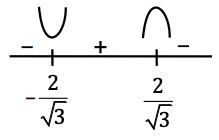Maximum area is possible at x = + 2 / √3 [put in eq.(1)]

Amax = 12 [2 / √3] - 3 (2 / √3)3

= [24 / √3] - [8 / √3] = 16 / √3 = 9.2

Question 10: Let f (x) = x|sin x|, x ∈ R. Then

1. a. f is differentiable for all x, except at x = n, n𝛑 = 1, 2, 3,….
2. b. f is differentiable for all x, except at x = n, n𝛑 = ±1, ± 2, ± 3,….
3. c. f is differentiable for all x, except at x = n, n𝛑 = 0, 1, 2, 3,….
4. d. f is differentiable for all x, except at x = n, n𝛑 = 0, ± 1, ± 2, ± 3,….

Solution:

f (x) = x|sin x|, x ∈ R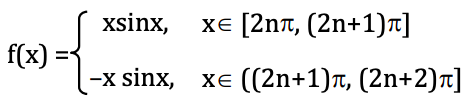Critical points are x = n𝛑

On differentiating f (x) with respect to x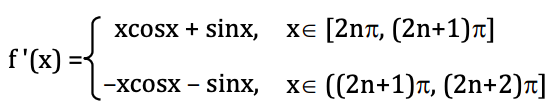LHD|x=(2n+1)𝛑 = (2n + 1)𝛑 . cos (2n + 1)𝛑 + sin (2n + 1)𝛑; n ≠ 0

= (2n + 1) 𝛑 (-1) = - (2n + 1)𝛑

RHD|x=(2n+1)𝛑 = - (2n + 1)𝛑 . cos (2n + 1)𝛑 - sin (2n + 1)𝛑

= (2n + 1)𝛑

LHD ≠ RHD ∀ x ∈ (2n + 1)𝛑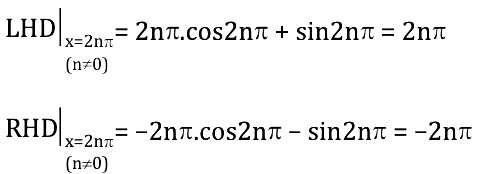LHD ≠ RHD ∀ x ∈ 2n𝛑 - {0}

LHD|x=0 = 0 × cos0 + sin0 = 0

RHD|x=0 = - 0 × cos0 - sin0 = 0

LHD = RHD for x = 0

From here we can say f(x) is differentiable ∀ x ∈ R, except x = n𝛑, where n = ± 1, ± 2, ± 3,….

Question 11: Let f: [–1, 1] → R be a function defined by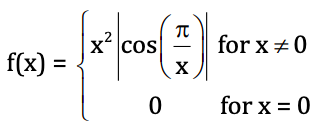The set of points where f is not differentiable is

1. a. {x ∈ [–1, 1]; x ≠ 0}
2. b. {x ∈ [–1, 1]; x = 0 or x = 2 / [2n + 1], n ∈ Z}
3. c. {x ∈ [–1, 1]; x = 2 / [2n + 1], n ∈ Z}
4. d. [–1, 1]

Solution: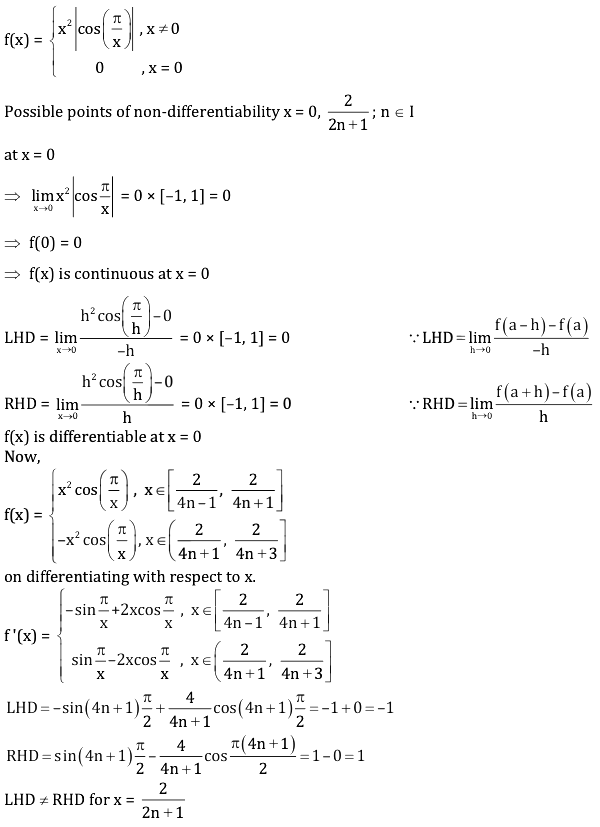Question 12: The value of the integral ∫0𝛑 (1– |sin 8x|) dx is

1. a. 0
2. b. 𝛑 – 1
3. c. 𝛑 – 2
4. d. 𝛑 – 3

Solution:

0𝛑 (1– |sin 8x|) dx

= ∫0𝛑 1 dx - ∫0𝛑 |sin 8x| dx

Using ∫0nT f (x) dx = n ∫0T f (x) dx

= x|0𝛑 - 8 ∫0𝛑/8 sin 8x dx [As |sin 8x| is periodic with period 𝛑 / 8]

= 𝛑 – 8 [- cos8x / x]0𝛑/8

= 𝛑 – 8 [(1 / 8) + (1 / 8)]

= 𝛑 – 8 (2 / 8)

= 𝛑 – 2

Question 13: Let ln (x) denote the logarithm of x with respect to the base e. Let S ⊂ R be the set of all points where the function ln(x2 – 1) is well-defined. Then the number of functions f: S → R that are differentiable, satisfy f ’(x) = ln (x2 – 1) for all x ∈ S and f (2) = 0, is

1. a. 0
2. b. 1
3. c. 2
4. d. infinite

Solution:

f’(x) = ln(x2 – 1) (Given)

f’(x) = ln(x + 1)(x – 1) {using A2 – B2 = (A + B)(A – B)}

f’(x) = ln(x + 1) + ln(x – 1) {using lnM.N = lnM + lnN}

On integrating both sides

f (x) = [ln(x + 1) ln(x – 1)]dx

Because ∫lnxdx = xlnx – x + C

f(x) = (x + 1) ln (x + 1) – (x + 1) + (x – 1) ln (x – 1) – (x – 1) + C

f (x) = x ln(x2 – 1) + ln [x + 1] / [x - 1] – 2x + C …(1)

put x = 2 in equation (1)

f (2) = 2 ln 3 + ln (3 / 1) – 4 + C = 0

C = 4 – 3ln3 [put in equation(1)]

f (x) = xln(x2 – 1) +ln [x + 1] / [x - 1] – 2x + 4 – 3ln3

Infinite C values possible in set S, then the number of functions in set S are infinite.

Question 14: Let S be the set of real numbers p such that there is no nonzero continuous function f: R → R satisfying ∫0x f (t) dt = p f (x) for all x ∈ R. Then S is:

1. a. the empty set
2. b. the set of all rational numbers
3. c. the set of all irrational numbers
4. d. the whole set R

Solution:

0x f (t) dt = p f (x) …(1)

Put x = 0 in eq.(1)

f (0) = 0 …(2)

On differentiating eq.(1)

p f’ (x) = f (x)

f '(x) / f (x) = 1 / p

On integrating both sides

ln f (x) = x / p + C

f (x) = Kex/p

f (0) = 0

f (0) = Ke0 = 0

K = 0

So, f (x) = 0

Hence S ∈ R

Question 15: The probability of men getting a certain disease is 1 / 2 and that of women getting the same disease is 1 / 5. The blood test that identifies the disease gives the correct result with probability 4 / 5. Suppose a person is chosen at random from a group of 30 males and 20 females, and the blood test of that person is found to be positive. What is the probability that the chosen person is a man?

1. a. 75 / 107
2. b. 3 / 5
3. c. 15 / 19
4. d. 3 / 10

Solution: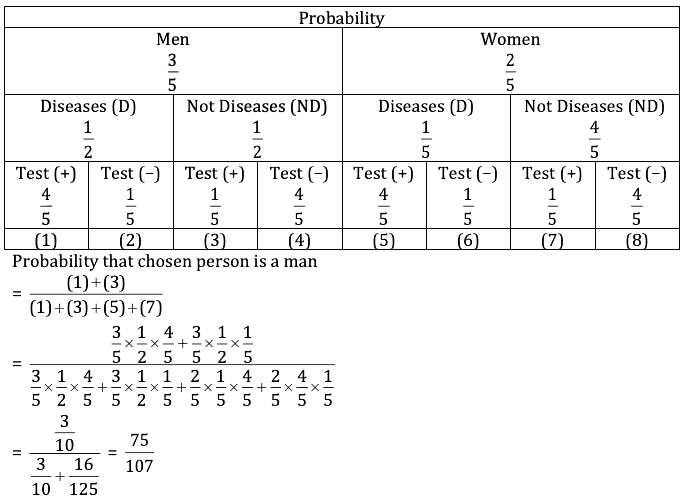Question 16: The number of functions f : [0, 1] → [0, 1] satisfying |f(x) – f(y)| = |x – y| for all x, y in [0, 1] is:

1. a. exactly 1
2. b. exactly 2
3. c. more than 2, but finite
4. d. infinite

Solution:

|f(x) – f(y)| = |x – y|

|f(x)– f(y)| / |x– y| = 1

Taking limit x → y

lim x→y |f(x) – f(y)| / |x– y| = 1

|f’(x)| = 1

f’(x) = ± 1

On integrating both sides

f (x) = ± x + C

f : [0, 1] → [0, 1]

f (x) = x or f (x) = 1 – x

Hence two functions possible.

Question 17: Suppose A is a 3 × 3 matrix consisting of integer entries that are chosen at random from the set {–1000, –999, …, 999, 1000}. Let P be the probability that either A2 = –I or A is a diagonal matrix, (where I is the 3 × 3 identity matrix). Then

1. a. P < 1 / 1018
2. b. P = 1 / 1018
3. c. 52 / 1018 ≤ P ≤ 53 / 1018
4. d. P ≥ 54 / 1018

Solution:

Given A = [ ]3×3 &

A2 = –I or diagonal matrix

If we take determinant both sides We get |A|2 = –1 (not possible) then A is a diagonal matrix

$\begin{bmatrix} ? & 0 &0 \\ 0& ? &0 \\ 0 &0 & ? \end{bmatrix}$

Total matrices = (2001)9{Each of 9 elements can take 2001 values from given set} Favourable cases = (2001)3 {Each of 3diagonal elements can take 2001 values from given set}

P = 3 / 9 = 1 / 6 < 1 / 6 = 1 / 1018

P < (1 / 1018)

Question 18: Let xk be real numbers such that xk ≥ k4 + k2 + 1 for 1 ≤ k ≤ 2018. Denote N = ∑k=12018 k. Consider the following inequalities: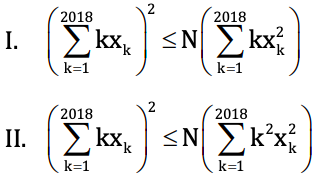Then

1. a. both I and II are true
2. b. I is true and II is false
3. c. I is false and II is true
4. d. both I and II are false

Solution:

If x1, x2, …., xn be n numbers then using Cauchy-Schwarz theorem

Case (i) consider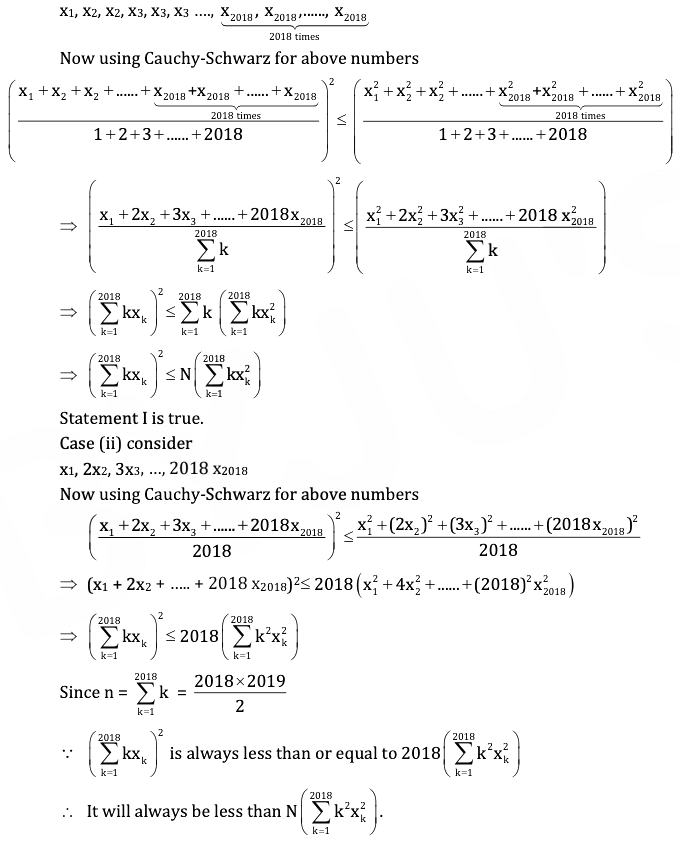Question 19: Let x2 = 4ky, k > 0, be a parabola with vertex A. Let BC be its latus rectum. An ellipse with centre on BC touches the parabola at A and cuts BC at points D and E such that BD = DE = EC (B, D, E, C in that order). The eccentricity of the ellipse is

1. a. 1 / √2
2. b. 1 / √3
3. c. √5 / 3
4. d. √3 / 2

Solution: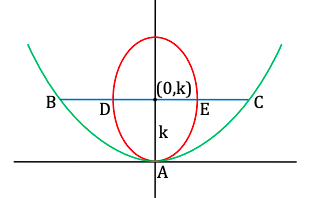x2 = 4ky

Because 4a = 4k

a = k

BD = DE = EC

DE = BC / 3 = 4k / 3

DE = 2b = 4k / 3

b = 2k / 3

e = √1 - (b2 / a2)

= √1 - [4k2 / 9k2]

= √1 - (4 / 9)

= √5 / 3

Question 20: Let f: [0, 1] → [–1, 1] and g: [–1, 1] → [0, 2] be two functions such that g is injective and gof: [0, 1] → [0, 2] is surjective. Then

1. a. f must be injective but need not be surjective
2. b. f must be surjective but need not be injective
3. c. f must be bijective
4. d. f must be a constant function

Solution: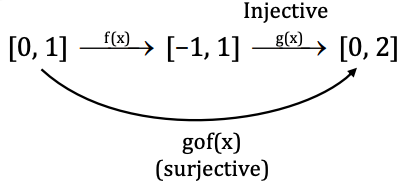f (x) should be surjective,

If f (x) is not surjective then it will get the whole co-domain [–1, 1] (which is the domain of g) and then consecutively gof would not be able to reach the whole [0, 2] (g is injective).

Question 21: Let R be a rectangle, C be a circle, and T be a triangle in the plane. The maximum possible number of points common to the perimeters of R, C, and T is

1. a. 3
2. b. 4
3. c. 5
4. d. 6

Solution: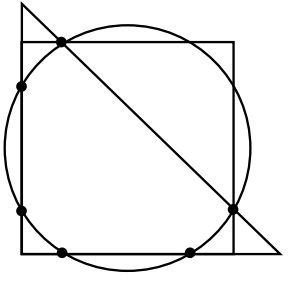Maximum number of common points = 6

Question 22: The number of different possible values for the sum x + y + z, where x, y, z are real numbers such that x4 + 4y4 + 16z4 + 64 = 32xyz is

1. a. 1
2. b. 2
3. c. 4
4. d. 8

Solution:

Given: x4 + 4y4 + 16z4 + 64 = 32xyz …(1)

Using AM ≥ GM

[x4 + 4y4 + 16z4 + 64] / 4 ≥ (x4 . 4y4 . 16z4 . 64)¼

32xyz / 4 ≥ 8xyz (from eq.(1))

8xyz ≥ 8xyz

AM = GM

x4 = 4y4 = 16z4 = 64

x4 = 64, 4y4 = 64, 16z4 = 16

x = ± 2 √2, y = ± 2, z = ± √2

Possible cases for sums, when we know 32xyz are positive.

(2√2, 2, √2), (–2 √2, –2, √2), (2 √2, –2, – √2), (–2 √2, 2, – √2)

Hence, the number of possible values for sum x + y + z are 4.

Question 23: Let Γ be a circle with diameter AB and centre O. Let l be the tangent to Γ at B. For each point, M on Γ different from A, consider the tangent t at M and let it intersect l at P. Draw a line parallel to AB through P intersecting OM at Q. The locus of Q as M varies over Γ is

1. a. an arc of a circle
2. b. a parabola
3. c. an arc of an ellipse
4. d. a branch of a hyperbola

Solution: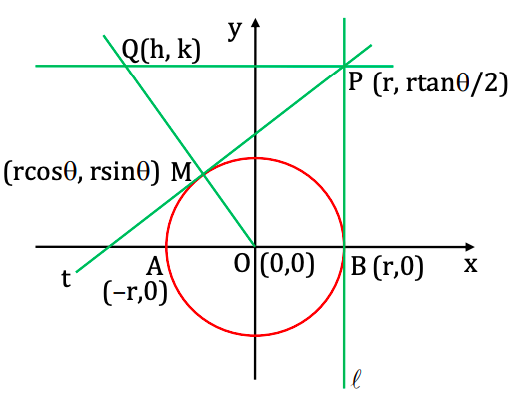Let equation of circle x2 + y2 = r2 …(1)

Let point M on circle (r cos θ, r sin ) {using parametric coordinate of circle} Equation of tangent at M is x cos θ + y sin θ = r …(2) {Using T = 0 xx1 + yy1 = r2}

at x = r, y = r (1 - cos θ) / sin θ

Coordinate of P (r, r (1 - cos θ) / sin θ) (using equation (2))

P ≡ (r, r tan [θ / 2]) {1 – cos θ = 2sin2 θ / 2 ; sin θ = 2sin θ / 2 cos θ / 2}

Equation of OM

(y – 0) = [rsinθ – 0] / [rcosθ – 0] {x – 0} {using y - y1= m (x - x1); m = (y2 - y1) / (x2 - x1)

y = x tan θ…(3)

Let Q = (h, k)

Q (h, k) satisfies equation (3)

k = h tan θ…(4)

k = height of point P from x-axis & AB is parallel to PQ

k = r tan θ / 2 (put in equation 4)

h =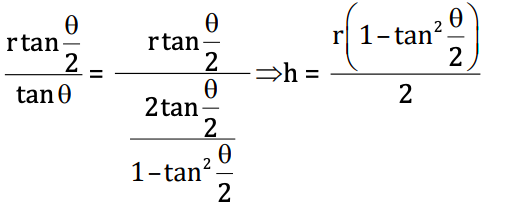Using value of h & k

h = (r / 2) (1 - [k / r]2) {Since tan θ / 2 = k / r}

(2h / r) = 1 - [k2 / r2]

2hr = r2 – k2

Required locus is 2rx = r2 – y2 (Equation of parabola)

Question 24: The number of solutions x of the equation sin (x + x2) – sin (x2) = sinx in the interval [2, 3] is

1. a. 0
2. b. 1
3. c. 2
4. d. 3

Solution:

Given: sin (x + x2) – sin (x2) = sinx

Using sin C – sin D = 2 cos [C + D] / 2 sin [C - D] / 2 & sinx = 2 sin (x / 2) cos (x / 2)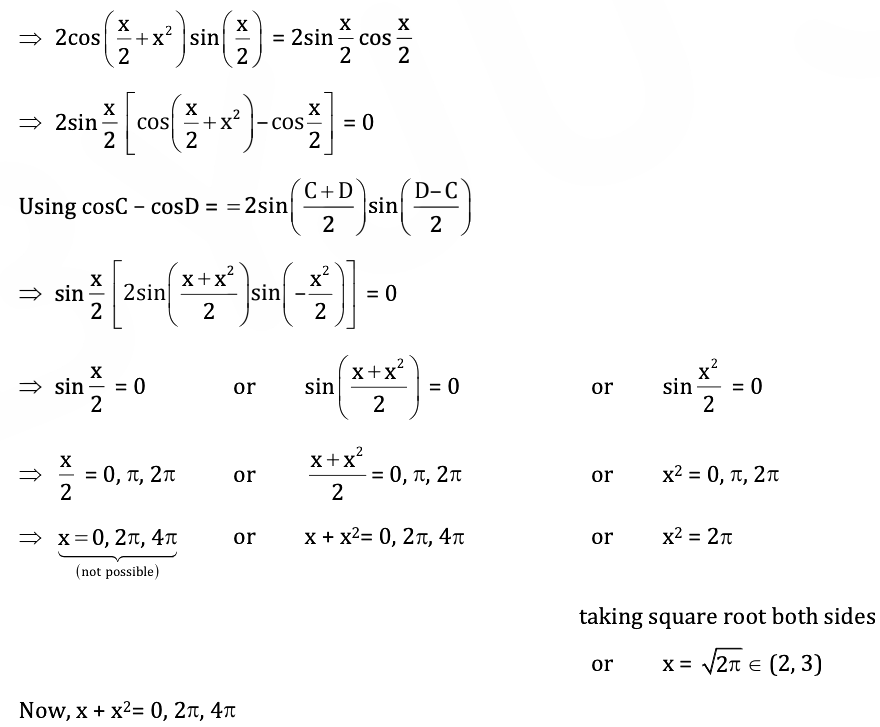Case: I

x + x2 = 0

x(x + 1) = 0

x = 0 or x = –1 ∉ 2, 3 [not possible]

Case: II

or x + x2 = 2𝛑

or x + x2 – 2𝛑 = 0

= [-1 ± √1 + 8𝛑] / 2

= [-1 ± 5.11] / 2

x = -6.1 / 2 ∉ (2, 3)

x = 4.11 / 2 = 2.05

x = 2.05 ∈ (2, 3)

Case: III

x + x2 = 4𝛑

or x + x2 – 4𝛑 = 0

= [-1 ± √1 + 16𝛑] / 2

= [-1 ± 7.08] / 2

x = ([-8.08 / 2], [6.08 / 2])

x = -4.04, 3.01 ∉ (2, 3) [not possible]

Total number of solution lies between (2, 3) = 2

Question 25: The number of polynomials P: R → R satisfying P(0) = 0, P(x) > x2 for all x ≠ 0, and P”(0) = 1 / 2 is:

1. a. 0
2. b. 1
3. c. more than 1, but finite
4. d. infinite

Solution:

Let f(x) = p(x) – x2 …(1) [Because p(x) is polynomial, f (x) is also polynomial] p(x) > x2 (given)

p(x) – x2 > 0 …(2)

from (1) & (2)

f (x) > 0 ∀ x ≠ 0 …(3)

but

f (0) = p (0) – 0 = 0 …(4) [Because p(0) = 0 (given)]

Using conditions of eq.(3) & (4) we can say, x = 0 should be a point of minima and f"(x) should be greater than or equal to 0.

f"(x) ≥ 0 at x = 0 (using concept of concavity)

Now,

f(x) = p(x) – x2

on differentiating with respect to x.

f'(x) = p'(x) – 2x

again differentiating with respect to x.

f"(x) = p"(x) – 2

Put x = 0 in f"(x)

f"(0) = p"(0) – 2

= (1 / 2) – 2 [Because p"(0) = 1 / 2 (given)]

= – 3 / 2

f"(x) = – 3 / 2 < 0

f"(x) < 0 (which is contradict)

No such polynomials exist.

Question 26: Suppose the limit L = limx→∞ √n ∫01 1 / [1 + x2]n dx, exists and is larger than 1 / 2. Then

1. a. 1 / 2 < L < 2
2. b. 2 < L < 3
3. c. 3 < L < 4
4. d. L ≥ 4

Solution: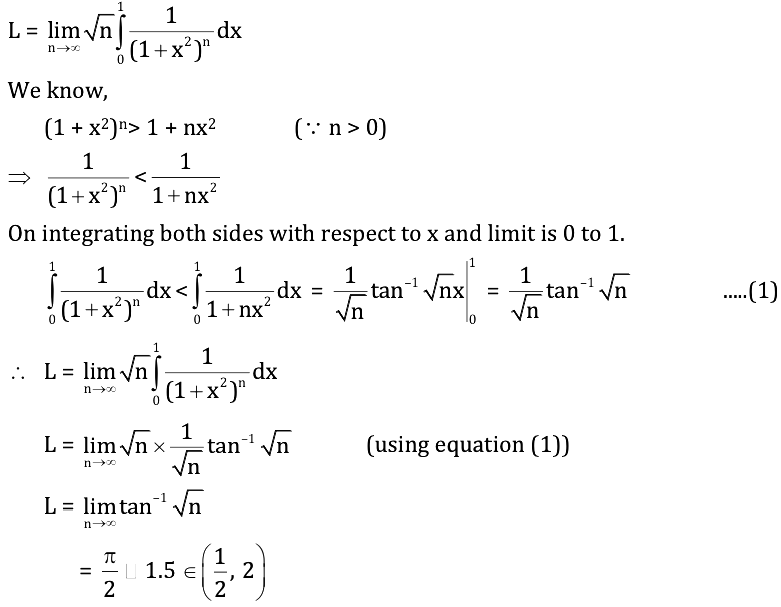Question 27: Consider the set An of points (x, y) such that 0 ≤ x ≤ n, 0 ≤ y ≤ n where n, x, y are integers. Let Sn be the set of all lines passing through at least two distinct points from An. Suppose we choose a line l at random from Sn. Let Pn be the probability that l is tangent to the circle x2 + y2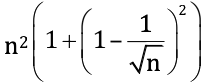.

Then the limit limx→∞ Pn is

1. a. 0
2. b. 1
3. c. 1 / 𝛑
4. d. 1 / √2

Solution:

A total number of points in set:

An = (n + 1)2

The total number of lines:

Sn = (n+1)^2C2

Equation of circle:

x2 + y2 =[Given]

Let the number of tangents possible is K.

Then Probability Pn = Number of tangents / Total lines

= K / [(n+1)^2C2]

= K . 2![(n + 1) –2]! / (n + 1)2! [Because nCr = n! / [r! (n - r)!]

limx→∞ Pn = 0 (Power of n in the denominator is greater than numerator)

Question 28: Let f:[0, 1] → R be an injective continuous function that satisfies the condition –1 < f (0) < f (1) < 1 Then the number of functions g: [–1, 1] → [0, 1] such that (g o f) (x) = x for all x ∈ [0, 1] is

1. a. 0
2. b. 1
3. c. more than 1, but finite
4. d. infinite

Solution: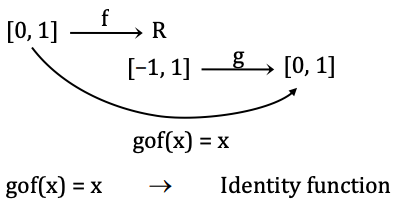For defining g o f (x) = x ∀ x ∈ [0, 1], g (x) should attain all values in [0, 1] when range of f (x) a subset of (–1, 1) is used as image for g(x).

There may be an infinite number of such f’s and a correspondingly infinite number of g’s.

Question 29: The maximum possible area bounded by the parabola y = x2 + x + 10 and a chord of the parabola of length 1 is

1. a. 1 / 12
2. b. 1 / 6
3. c. 1 / 3
4. d. 1 / 2

Solution:

y = x2 + x + 10

Vertex ≡ [(-b / 2a), (-D / 4a)] ≡ [(-1 / 2), (39 / 4)]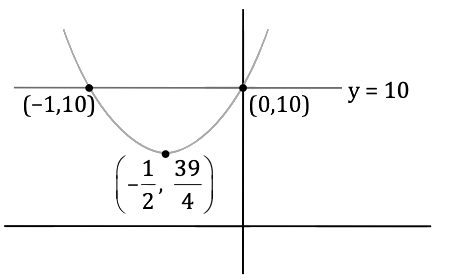Let (x1, y1) and (x2, y2) are extreme points of the chord on parabola.

Distance between points = 1 (given)

(x1 – x2)2 + (y1 – y2)2 = 1 …(1)

Point are on parabola,

y1 = x12 + x1 + 10 …(2)

y2 = x22 + x2 + 10 …(3)

Eq.(2) – Eq.(3)

y1 – y2 = x12 – x22 + x1 – x2

y1 – y2 = (x1 – x2) (x1 + x2 + 1)

Put in eq.(1), then,

(x1 – x2)2 [1 + (x1 + x2 + 1)2] = 1

x1 – x2 = 1 or x1 + x2 + 1 = 0

On solving both equation we get x1 = 0 & x2 = –1

Put in eq.(2) & (3)

y1 = 10 & y2 = 10

So, points are (x1, y1) = (0, 10)

(x2, y2) = (–1, 10)

The maximum area will be bounded by horizontal chords.

A = ∫-10 [10 - (x2 + x + 10)] dx

= ∫-10 (-x2 - x) dx

= (-x3 / 3) - (x2 / 2)-10

= 0 - [(1 / 3) - (1 / 2)] = 1 / 6

Question 30: Suppose z is any root of 11z8 + 20iz7 + 10iz – 22 = 0, where i = √–1 . Then S = |z|2 + |z| + 1 satisfies

1. a. S ≤ 3
2. b. 3 < S < 7
3. c. 7 ≤ S <13
4. d. S ≥ 13

Solution:

11z8 + 20iz7 + 10iz – 22 = 0 (Given)

(11z8 – 22) + i (20z7 + 10z) = 0 = 0 + 0i

On comparing both sides

z8 = 22 / 11 or z7 = -10z / 20

z8 = 2 or z6 = -1 / 2

z6 . z2 = 2

(-1 / 2) z2 = 2 [Because z6 = -1 / 2]

z2 = -4

Taking square root both sides

z = ± 2i

Taking modulus both sides

|z| = 2

Then

S = |z|2 + |z| + 1

= 4 + 2 + 1 = 6 ∈ (3, 7)

### KVPY SX 2018 Maths Paper with Solutions